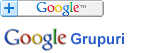Căutare în Webster - Dicționarul explicativ al limbii engleze

 Cuvânt
Pentru căutare rapidă introduceți minim 3 litere.

UNIT - Definiția din dicționar

Notă: Puteţi căuta fiecare cuvânt din cadrul definiţiei printr-un simplu click pe cuvântul dorit.

U"nit (?), n. [Abbrev. from unity.] 1.
[1913 Webster]

2. (Arith.)
[1913 Webster]

Units are the integral parts of any large number. I. Watts.
[1913 Webster]

3. Camden.
[1913 Webster]

4.
[1913 Webster]

5. (Math.)
[1913 Webster]

Abstract unit, the unit of numeration; one taken in the abstract; the number represented by 1. The term is used in distinction from concrete, or determinate, unit, that is, a unit in which the kind of thing is expressed; a unit of measure or value; as 1 foot, 1 dollar, 1 pound, and the like. -- Complex unit , an imaginary number of the form a + b-1, when a2 + b2 = 1. -- Duodecimal unit, a unit in the scale of numbers increasing or decreasing by twelves. -- Fractional unit, the unit of a fraction; the reciprocal of the denominator; thus, 1/4 is the unit of the fraction 3/4. -- Integral unit, the unit of integral numbers, or 1. -- Physical unit, a value or magnitude conventionally adopted as a unit or standard in physical measurements. The various physical units are usually based on given units of length, mass, and time, and on the density or other properties of some substance, for example, water. See Dyne, Erg, Farad, Ohm, Poundal, etc. -- Unit deme (Biol.), a unit of the inferior order or orders of individuality. -- Unit jar (Elec.), a small, insulated Leyden jar, placed between the electrical machine and a larger jar or battery, so as to announce, by its repeated discharges, the amount of electricity passed into the larger jar. -- Unit of heat (Physics), a determinate quantity of heat adopted as a unit of measure; a thermal unit (see under Thermal). Water is the substance generally employed, the unit being one gram or one pound, and the temperature interval one degree of the Centigrade or Fahrenheit scale. When referred to the gram, it is called the gram degree. The British unit of heat, or thermal unit, used by engineers in England and in the United States, is the quantity of heat necessary to raise one pound of pure water at and near its temperature of greatest density (39.1° Fahr.) through one degree of the Fahrenheit scale. Rankine. -- Unit of illumination, the light of a sperm candle burning 120 grains per hour. Standard gas, burning at the rate of five cubic feet per hour, must have an illuminating power equal to that of fourteen such candles. -- Unit of measure (as of length, surface, volume, dry measure, liquid measure, money, weight, time, and the like), in general, a determinate quantity or magnitude of the kind designated, taken as a standard of comparison for others of the same kind, in assigning to them numerical values, as 1 foot, 1 yard, 1 mile, 1 square foot, 1 square yard, 1 cubic foot, 1 peck, 1 bushel, 1 gallon, 1 cent, 1 ounce, 1 pound, 1 hour, and the like; more specifically, the fundamental unit adopted in any system of weights, measures, or money, by which its several denominations are regulated, and which is itself defined by comparison with some known magnitude, either natural or empirical, as, in the United States, the dollar for money, the pound avoirdupois for weight, the yard for length, the gallon of 8.3389 pounds avoirdupois of water at 39.8° Fahr. (about 231 cubic inches) for liquid measure, etc.; in Great Britain, the pound sterling, the pound troy, the yard, or 1/108719 part of the length of a second's pendulum at London, the gallon of 277.274 cubic inches, etc.; in the metric system, the meter, the liter, the gram, etc. -- Unit of power. (Mach.) See Horse power. -- Unit of resistance. (Elec.) See Resistance, n., 4, and Ohm. -- Unit of work (Physics), the amount of work done by a unit force acting through a unit distance, or the amount required to lift a unit weight through a unit distance against gravitation. See Erg, Foot Pound, Kilogrammeter. -- Unit stress (Mech. Physics), stress per unit of area; intensity of stress. It is expressed in ounces, pounds, tons, etc., per square inch, square foot, or square yard, etc., or in atmospheres, or inches of mercury or water, or the like.
[1913 Webster]